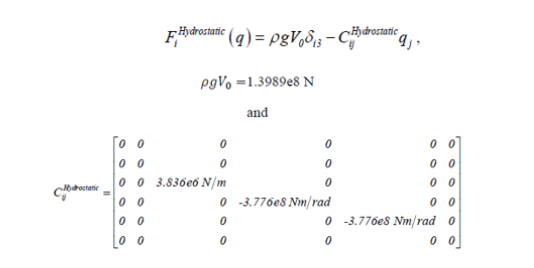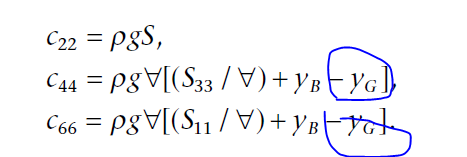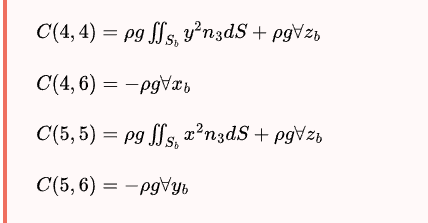# Hydrostatic restoring matrix

Hi Jason,
I am a bit confused about the hydrostatic restoring matrix. In the manual, it is highlighted that wamit only calculate the hydrostatic restoring provided by buoyancy, because gravatitional restoring would be intrinsicly considered in FAST in elastodyn. At the same time, the .hst file is a 6*6 matrix. This matrix seems exactly equal to the Cij in the picture?I feel that this part is the change of buoyancy, rather than the net buoyancy. So the rougVo is omitted here because it would be balanced by the gravity? And the Cij here is also the final hydrostatic restoring matrix in the equation of motion? I tried to solve the equation using wamit output file, and got confused about the matrix. I check the theory about hydrostatic in Newman’s book and find the expression. For the specific application in terms of FAST and WAMIT, i get lost.

Thanks
Lulu

Dear @Lulu.Liu,

I agree that the WAMIT .hst file to be used in HydroDyn should equal [C_ij]^Hydrostatic from your first equation. And I agree that this restoring matrix should not include the effects of body weight because body weight is intrinsically included in the structural modules of OpenFAST (ElastoDyn, SubDyn, BeamDyn), which would otherwise be double counted if included in [C_ij]^Hydrostatic. This restoring matrix will be different than the restoring matrix in your last equation (from Newman) because the restoring in your last equation must include the contribution from both buoyancy and body weight–i.e., the total restoring.

The undisplaced buoyancy term (rho * g * V_0) does not appear in your last equation (from Newman) because it should cancel with the weight of the floating system and vertical undisplaced mooring pretension.

Best regards,

Hi Jason
Thank you for your time and quick reply. I checked wamit manuual with the matrix. I was wondering the relationship between the C(i,j) in wamit mannual and the Cij^hydrostatic (that is the output file) in the defination of the semi-sub. Why there is an additional term (rho * g * V_0) ? This term is the most confusing one.
If I want to change the Cij from .hst file to the equation of motion (considering the contribution from weight), I just need to add the term -mg*Zg back to the element. Is that right? Because we assume Xg,Yg,Zg=0 in wamit. In this case, the structure is symmetry(Xg=Yg=0) and regardless of the different mode sequence.Best regards

Dear @Lulu.Liu,

The term rho * g * V_0 is not part of the hydrostatic restoring matrix. This is the undisplaced buoyancy of the body. That is, the total contribution from buoyancy is rho * g *V_0 - [C_ij]^Hydrostatis * q_j, as in your first equation. Often the rho * g * V_0 term is neglected because it is assumed to cancel with the body weight and vertical mooring pretension. In OpenFAST, however, the rho * g * V_0 term is included in HydroDyn because the body weight and vertical mooring pretension are present in other modules.

Regarding the z_g term, I agree that you can add that term back into the restoring matrix if you need the total restoring matrix.

Best regards,

Dear Jason
Many thanks. It is very helpful.

Regards

Dear Jonkman

For the lastest version (v3.4.1), my explanation of the hydrostatic restorating matrix is as follows. Please correct me if something is wrong.

1. Buoyancy and rotational buoyancy is considered in `p%F_HS_Moment_Offset` in WAMIT
``````  do iBody = 1, p%NBody
p%F_HS_Moment_Offset(1,iBody) = 0.0_ReKi
p%F_HS_Moment_Offset(2,iBody) = 0.0_ReKi
p%F_HS_Moment_Offset(3,iBody) =  InitInp%RhoXg*InitInp%PtfmVol0(iBody)
p%F_HS_Moment_Offset(4,iBody) =  InitInp%RhoXg*InitInp%PtfmVol0(iBody)*( InitInp%PtfmCOByt(iBody) - InitInp%PtfmRefyt(iBody)  )
p%F_HS_Moment_Offset(5,iBody) = -InitInp%RhoXg*InitInp%PtfmVol0(iBody)*( InitInp%PtfmCOBxt(iBody) - InitInp%PtfmRefxt(iBody)  )
p%F_HS_Moment_Offset(6,iBody) = 0.0_ReKi
end do
``````

Part of m·g (steel weight) is subtracted in ElastDyn, and another part (ballast weight) is considered in Morison Strip-Theory.

When VCG is specified as zero in frequency-domin WAMIT, moments caused by weight are considered in corresponding modules. And, where C(4,6) and C(5,6) are generally zero.

1. According to Moment of inertia, data interpolation in OpenFast, linear added mass matrix, linear hydrostatic matrix - #2 by Jason.Jonkman, HydroDyn inputs `PtfmRefxt` , `PtfmRefyt` , and `PtfmRefzt` must be consistent with (0,0,VCG) in frequency-domain WAMIT because hydrostatic restoring matrix, exciting forces and added-mass and damping coefficients are related to (0,0,VCG).
In conjunction with the above discussion, `PtfmRefxt` , `PtfmRefyt` , and `PtfmRefzt` are always zero.

Dear @TianYuan.Wang,

• Hydrostatics in the potential-flow solution of HydroDyn is linear (as you summarize) and the matrix is an input to HydroDyn via the .hst file (typically calculated using WAMIT (or equivalent) in a preprocessing step).
• I generally agree with your linear hydrostatic restoring matrix except that the body mass terms (z_g) are not accounted for in HydroDyn; the body mass terms are accounted for in the structural modules, and so, should not be accounted for in HydroDyn. As such, when using WAMIT (or equivalent) in a preprocessing step, you should set VCG (=z_g) in WAMIT to zero.
• Regarding the use of `PtfmRefxt`, `PtfmRefyt`, `PtfmRefzt`, and `PtfmRefztRot`, I suggest referring to the following HydroDyn documentation: https://openfast.readthedocs.io/en/main/_downloads/2233f99533f60b1cca3448beeddcd02c/HydroDyn_Plan_TCF_NBody.docx.
• Hydrostatics in the strip-theory solution of HydroDyn is nonlinearly and analytically exact for members that are cylindrical or tapered cylinders.

Best regards,

Dear Jonkman

I found some related codes of mass forces in ElastDyn-SUBROUTINE CalculateForcesMoments:

But I didn’t find any information in ElastDyn about mgX_g, mgY_g, mgZ_g in the hydrostatic restoring matrix.

I think the reference point of the restoring matrix in WAMIT is (0,0,VCG) and that the restoring matrix will eventually multiply with the displacement at (0,0,VCG), and it is the same as wave radiation calculations (velocity and acceleration). Therefore, (PtfmRefxt, PtfmRefyt, PtfmRefzt) must completely correspond with (0,0,VCG).

If I use alternative WAMIT FORCE CONTROL FILE, which is able to specify the COG position, should I set (PtfmRefxt, PtfmRefyt, PtfmRefzt) = (XCG,YCG,ZCG) to match displacement multiplied by restoring matrix (and accereration multiplied by added mass matrix)?

Beat regards,

Dear @TianYuan.Wang,

The ElastoDyn source code snippet you show does calculate the contribution of the body mass term to the restoring. The calculation is not implemented as a restoring matrix times displacement, but the same effect is accounted for via the cross product in `TmpVec2`. The platform center of mass in ElastoDyn is specified via `PtfmCMxt`, `PtfmCMyt`, and `PtfmCMzt`, which are stored in `RtHSdat%rZY`.

All matrices output by WAMIT are relative to the origin of the coordinate system about which the panels are defined; this does not need to be (0,0,VCG). Moreover, the HydroDyn documentation (4.2.8.4. Modeling Considerations — OpenFAST v3.4.1 documentation) recommends setting VCG = 0 in WAMIT to avoid double counting the body mass effect in OpenFAST, as I noted above.

When using WAMIT, I would typically only use alternative form 2 of the FRC file when generating the second-order solution, in which case you must properly account for the FOWT mass matrix, center of gravity, and mooring system restoring matrix, as mentioned in the HydroDyn documentation I linked to above.

Best regards,

I got it. Many thanks!

Best regards,